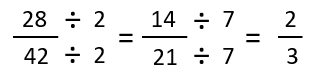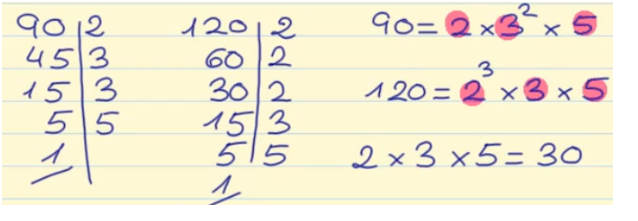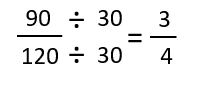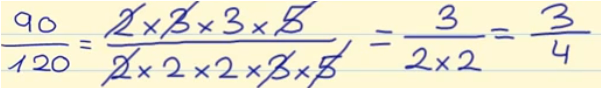Smartick is a fun way to learn math!Sep23

# How to Simplify Fractions: Lowest Terms Fractions

In this post, we are going to learn how we can calculate the lowest terms fractions by reducing them.
Before we start, let’s see what lowest terms fractions are: lowest terms fractions are fractions that cannot be simplified further.
How do we find the lowest terms of a fraction? There are two methods:

• Method 1: Divide the numerator and denominator by a common factor between them until there are no more common factors. Let’s look at an example:Let’s reduce the fraction 28/42. Both the numerator and denominator can be divided by 2. This would leave us at 14/21 and since 14 and 21 are multiples of 7, we can divide by 7. In dividing 14 and 21 by 7, we end up with 2/3, which is in the lowest terms since there is no longer a common factor between numerator and denominator.

• Method 2: Divide the numerator and denominator by the greatest common factor (GCF). Let’s see how we would reduce 90/120 with this method:We calculate the greatest common factor between 90 and 120. As shown above, we take the common prime factors of 90 and 120 (including repeats), which are 2, 3 and 5, and multiply them: 2 x 3 x 5 = 30.30 is the greatest common factor between 90 and 120. Therefore, we divide the numerator and denominator by 30. 90/120 written in lowest terms is 3/4.

Let’s look at a trick to put fractions in lowest terms. As shown in the image below, we will break down both the numerator (90) and the denominator (120) into prime factors and write the fraction with all of the factors. Cross out the factors found both in the numerator and denominator, and multiply the factors that are not crossed out. In the end, we have 3/4, which is the same result obtained using method 2.Fun is our brain’s favorite way of learning
Diane Ackerman
Smartick is a fun way to learn math
• 15 fun minutes a day